Question

Inorganic Chemistry

1. For the following questions you may need to look-up character tables online.

An object has a four-fold principle rotation axis. For the irreducible representationcontaining (x,y) in its linear functions column, use matrices to determine thecharacters in the columns headed by E, C4 and the next listed rotation operation.Also show how matrices can be used to determine the number in front of each ofthe operations i.e. the number of unique operations. Fully explain your reasoning.

For the Dsh character table explain the logic used to assign Mulliken symbols to the irreducible representations. You should ignore the subscripts 1 and 2 for the representations but explain all other parts of each Mulliken symbol.

Explain the relationship between the symmetry element i, the characters in the column headed by i in character tables and the subscripts g or u that are used in crystal field and molecular orbital energy level diagrams.Verified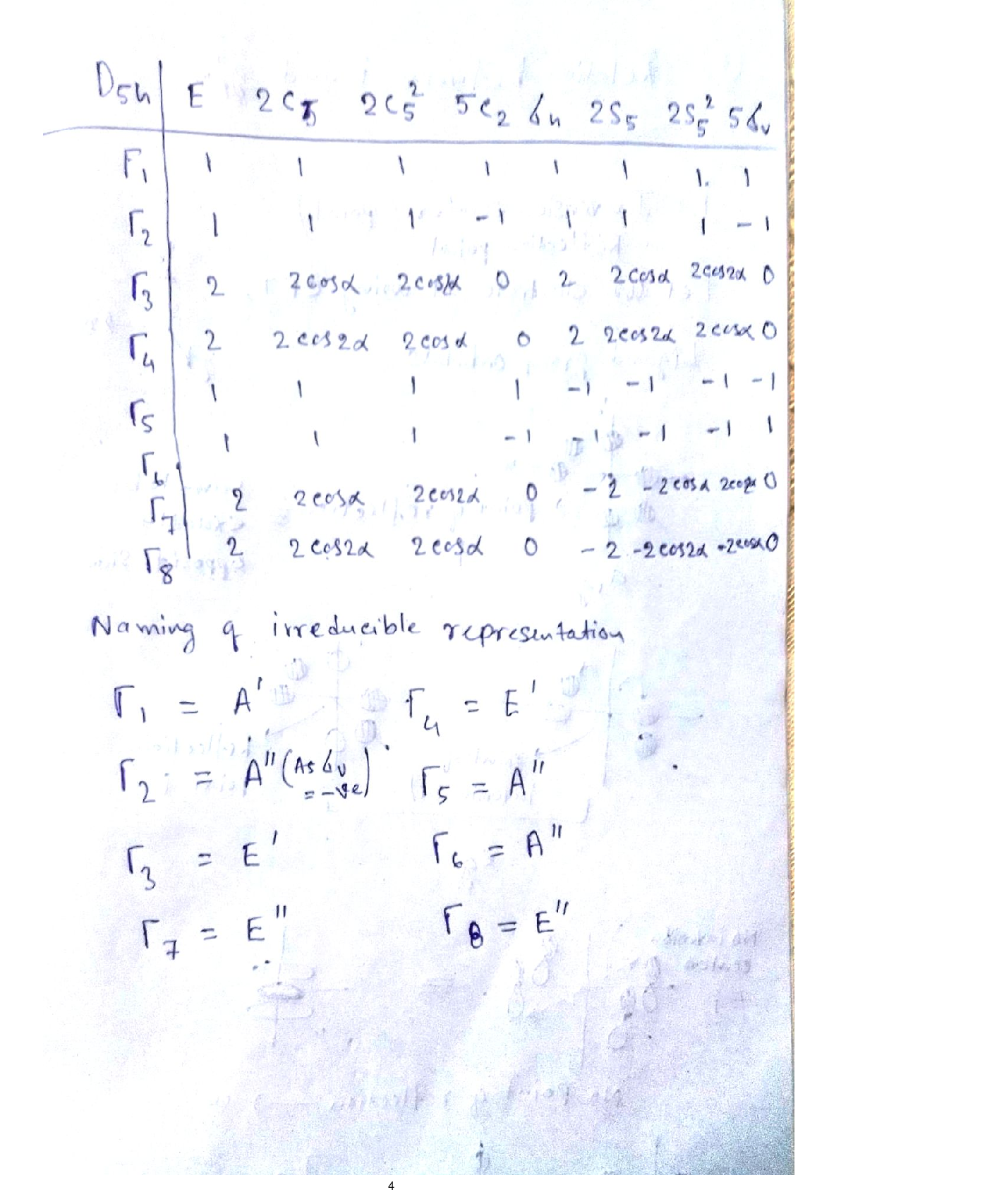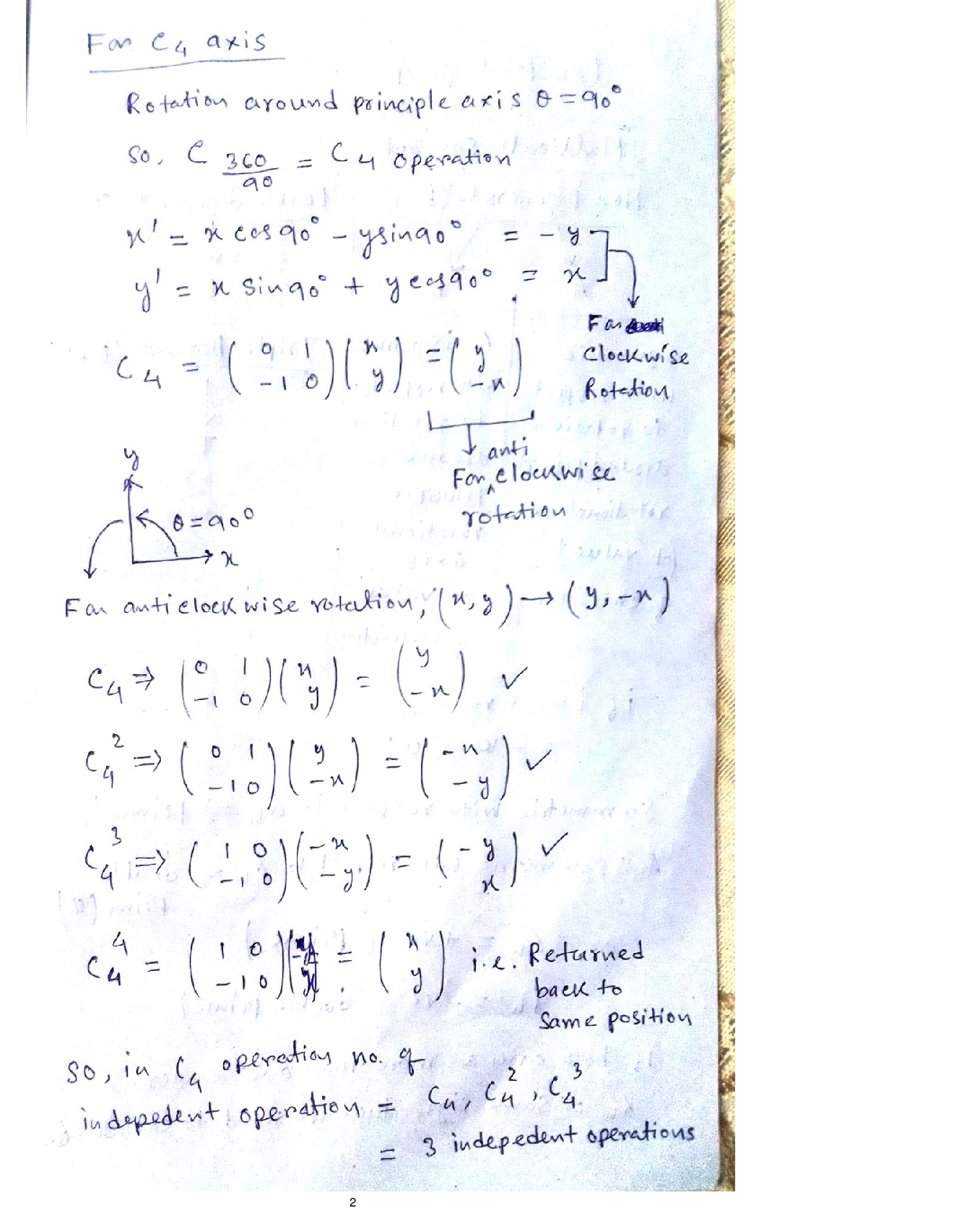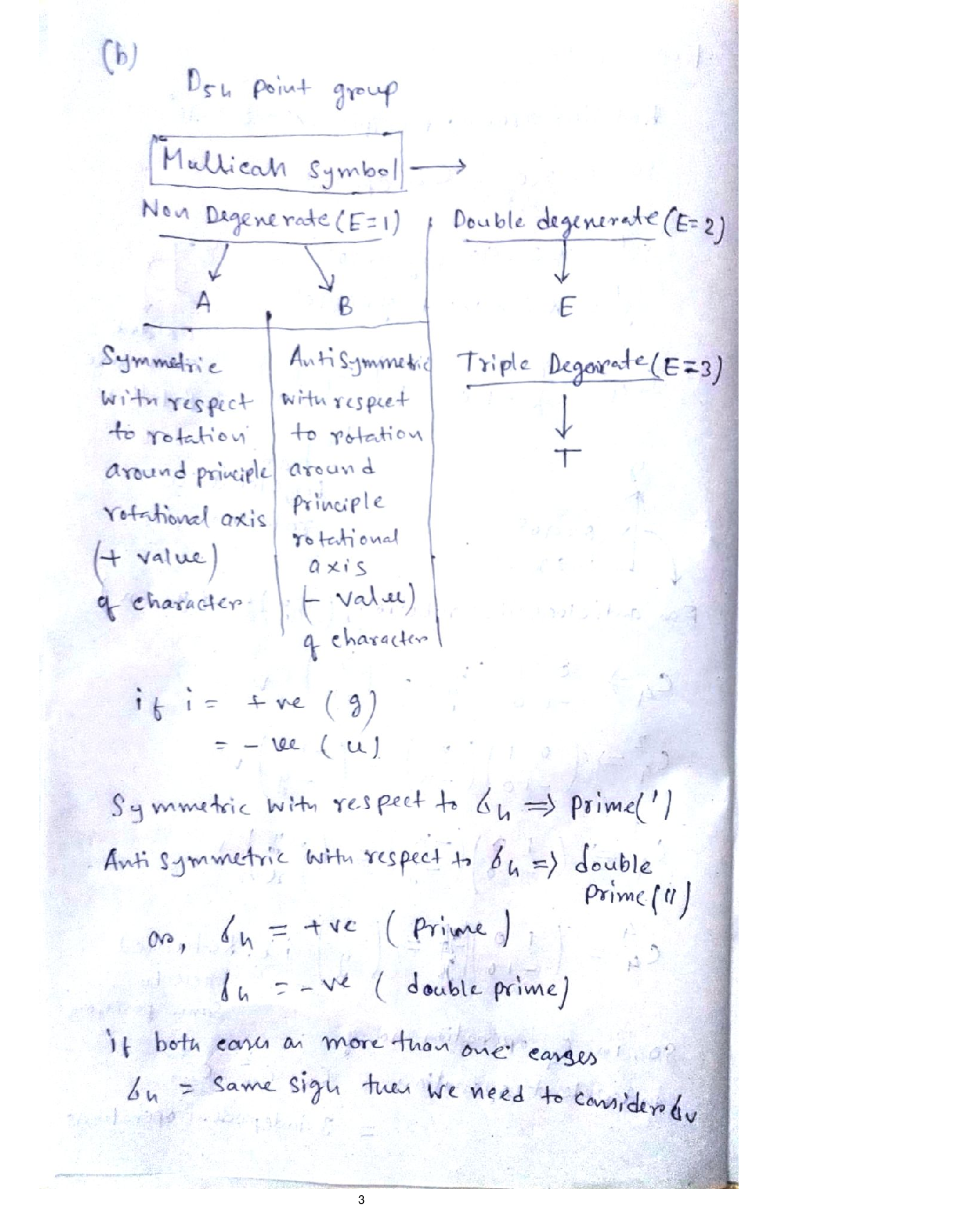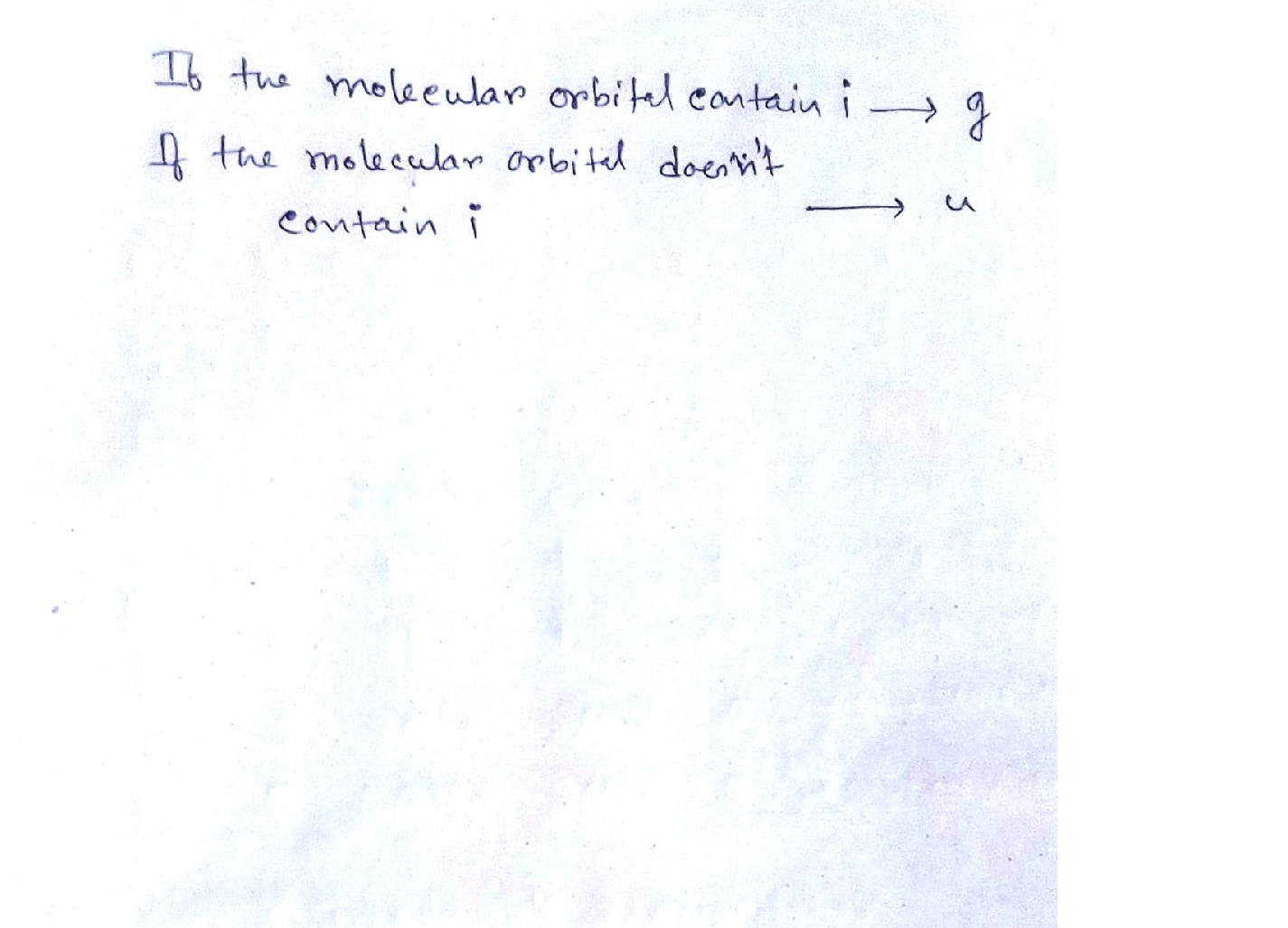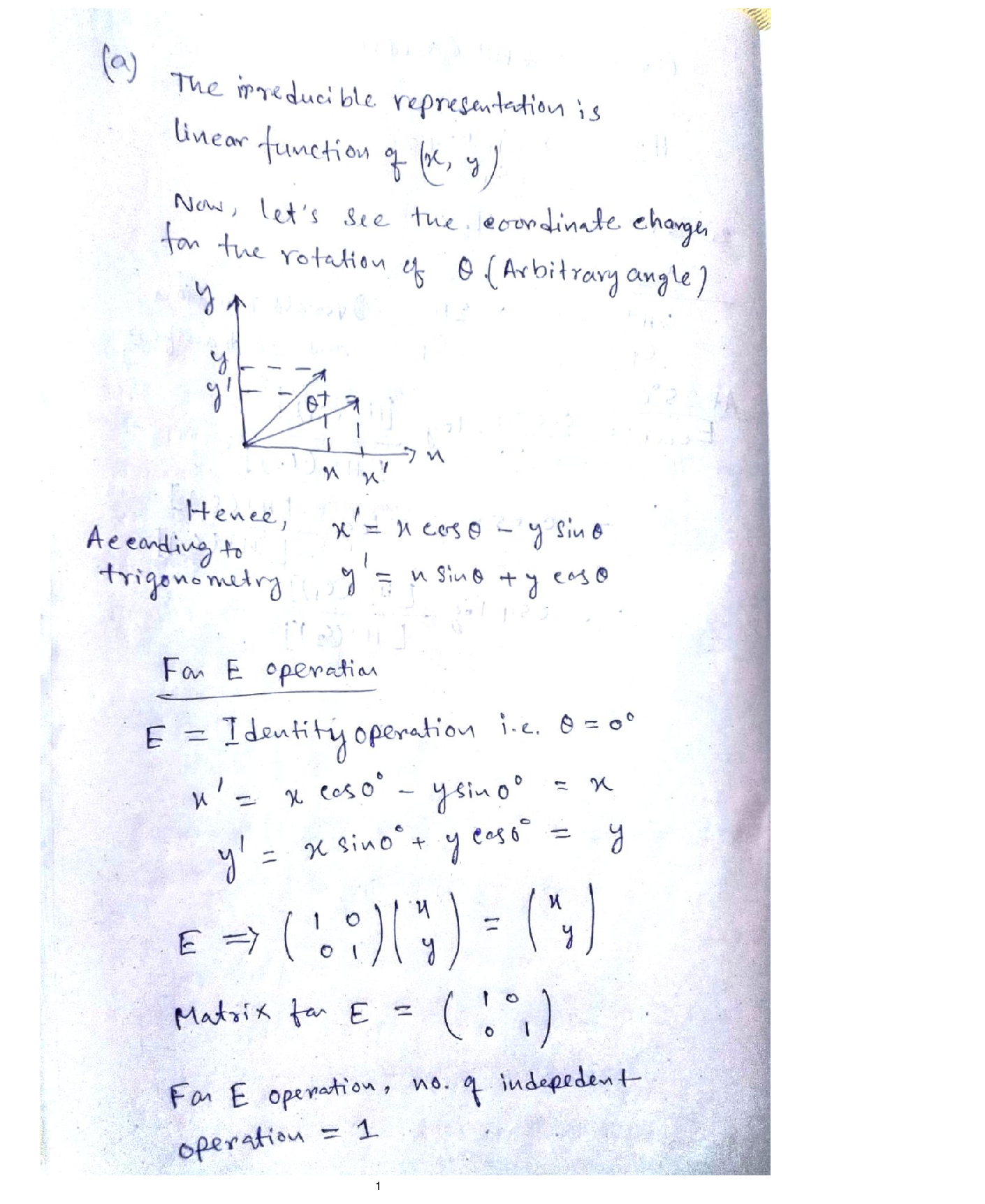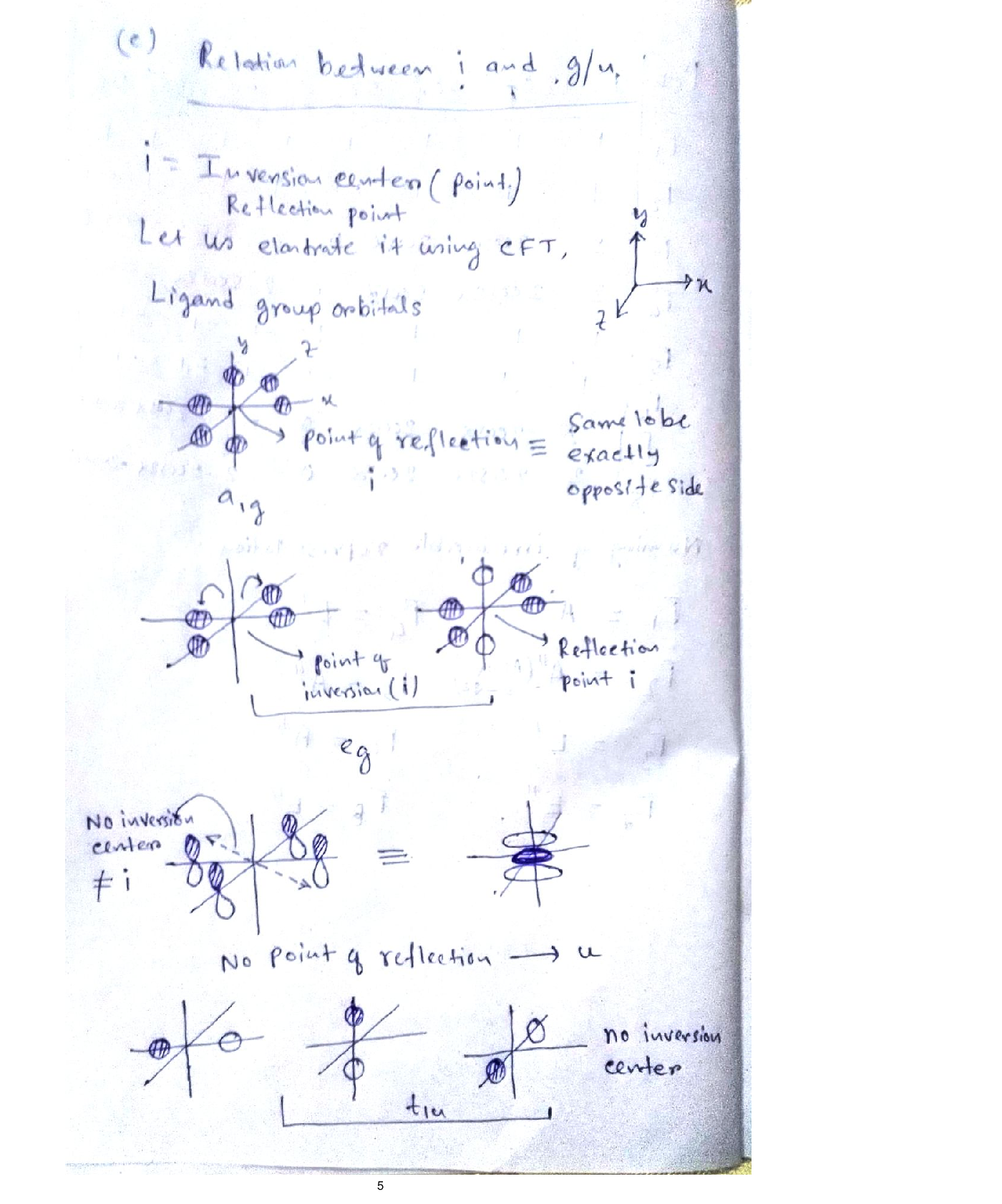### Question 43792Inorganic Chemistry

1. For the following questions you may need to look-up character tables online.
An object has a four-fold principle rotation axis. For the irreducible representationcontaining (x,y) in its linear functions column, use matrices to determine thecharacters in the columns headed by E, C4 and the next listed rotation operation.Also show how matrices can be used to determine the number in front of each ofthe operations i.e. the number of unique operations. Fully explain your reasoning.
For the Dsh character table explain the logic used to assign Mulliken symbols to the irreducible representations. You should ignore the subscripts 1 and 2 for the representations but explain all other parts of each Mulliken symbol.
Explain the relationship between the symmetry element i, the characters in the column headed by i in character tables and the subscripts g or u that are used in crystal field and molecular orbital energy level diagrams.

### Question 43679Inorganic Chemistry

The observed rate constant kobs for ligand substitution reactions at square planar platinum(II) complexes is a combination of the rate constants from competing pathways with (kı) and without (k2) solvent participation. The rate constant (k2) for the substitution of a chloride in trans-[Pt(py)2C12] by different ligands (Y) in methanol solvent at 30 °C and the nucleophilicity parameter (npi) of these ligands is given below:(c)
when Y = MeOH=solvent; the rate of reaction is 0.00027 s-'.
Use this data to calculate the nucleophilic discrimination factor (s) for the complex [Pt(py)2C12]. Show all of your working. (py = pyridine)
In methanol solvent at 30 °C the nucleophilic discrimination factor (s) for[Pt(dien)(OH2)]* = 0.44. Using your value of s from part (i) would you expect [Pt(dien)(OH2)]2* to be generally more or less reactive towards ligand substitution? Explain your answer.dien=diethylenetriamine H2N(CH2)2NH(CH2)2NH2)

### Question 43648Inorganic Chemistry

Consider the Frost diagram of some actinoids (An) in aqueous solution shown below:
Answer the following questions and fully explain your reasoning in each case.
Why does Bk show only 2 oxidation states while Am, Pu, U show 4 different oxidation states?
Why is the oxidation state +4 particularly stable for the element Th?
The oxocations AnO2* and AnO2²* (An = U, Pu, Am) have been isolated and studied. However, the oxocations LnO2+ and LnO2²+(Ln = Nd, Sm, Eu) areunknown. How can these observations be explained?
Would you expect the following molecules to be observed? Explain your answer.
CIF7
ArF4
Fully explain the origin of the difference in overall stability constants, measured in aqueous solution, for the two copper complexes in the table below.

### Question 38446Inorganic Chemistry

What is the ground state(A or E) for Octahedral d7,d8 and d9 ?:For an A ground state, a= 4. and for an E ground state, a= 2 Also Is it high or low spin? Give two examples for each of them (d7,d8and d9) and Calculate ueff ( like the example in the attachment)for each?
\mu \mathrm{eff}=\mu(\text { spin-only })(1-\alpha \lambda / \Delta o c t)

### Question 29224Inorganic Chemistry

I need an electron to be traveling at 0.970c. What potential difference should I use to accelerate it, assuming it starts from rest? Recall U=qV.

### Question 29223Inorganic Chemistry

An electron is accelerated from rest with acceleration a=5.0m/s2. How much energy has it lost after it has traveled a distance of 10m?t)

### Question 17215Inorganic Chemistry

3. Which would you expect to have a higher boiling point. A molecule with a large molecular dipole or a molecule with no molecular dipole. Explain your choice

### Question 17214Inorganic Chemistry

2. Can a molecule have bond dipoles but not have a molecular dipole? Explain.

### Question 17213Inorganic Chemistry

1. Which way do the bond arrows point?

### Question 17212Inorganic Chemistry

| Rotate the molecule to keep the "linear" arrangement (see below) and set the electronegativities of A, Band C to middle. Click to show the bond dipoles,molecular dipoles and partial charges.
Draw the bond dipoles on the bonds in the diagram
Show the overall molecular dipole in the diagram
Draw the partial charge symbols on the diagram.

### Submit query